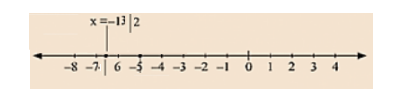# Give the geometrical representation of 2x + 13 = 0 as an equation in

Question:

Give the geometrical representation of 2x + 13 = 0 as an equation in

(i) one variable (ii) two variables

Solution:

We are given,

2x + 13 = 0

We get,

2x = -13

x = -13/2

The representation of the solution on the number line, when given equation is treated as an equation in one variable.The representation of the solution on the Cartesian plane, it is a line parallel to y axis passing through the point (-13/2, 0) is shown below.